# The MDC Procedure

### Multinomial Logit and Conditional Logit

Subsections:

When explanatory variables contain only individual characteristics, the multinomial logit model is defined as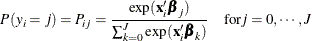whereis a random variable that indicates the choice made,is a vector of characteristics specific to the ith individual, and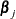is a vector of coefficients specific to the jth alternative. Thus, this model involves choice-specific coefficients and only individual specific regressors. For model identification, it is often assumed that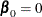. The multinomial logit model reduces to the binary logit model if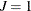.

The ratio of the choice probabilities for alternatives j and l (the odds ratio of alternatives j and l) is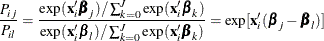Note that the odds ratio of alternatives j and l does not depend on any alternatives other than j and l. For more information, see the section Independence from Irrelevant Alternatives (IIA).

The log-likelihood function of the multinomial logit model is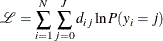where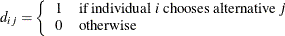This type of multinomial choice modeling has a couple of weaknesses: it has too many parameters (the number of individual characteristics times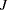), and it is difficult to interpret. The multinomial logit model can be used to predict the choice probabilities, among a given set of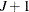alternatives, of an individual with known vector of characteristics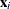.

The parameters of the multinomial logit model can be estimated with the TYPE=CLOGIT option in the MODEL statement; however, this requires modification of the conditional logit model to allow individual specific effects.

The conditional logit model, sometimes called the multinomial logit model, is similarly defined when choice-specific data are available. Using properties of Type I extreme-value (Gumbel) distribution, the probability that individual i chooses alternative j from among the choices in his choice set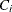is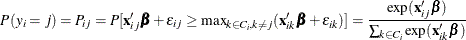where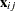is a vector of attributes specific to the jth alternative as perceived by the ith individual. It is assumed that there are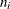choices in each individual’s choice set,.

The log-likelihood function of the conditional logit model is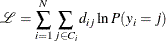The conditional logit model can be used to predict the probability that an individual will choose a previously unavailable alternative, given knowledge ofand the vectorof choice-specific characteristics.

#### Independence from Irrelevant Alternatives (IIA)

The problematic aspect of the conditional logit (and the multinomial logit) model lies in the property of independence from irrelevant alternatives (IIA). The IIA property can be derived from the probability ratio of any two choices. For the conditional logit model,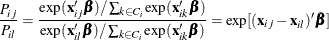It is evident that the ratio of the probabilities for alternatives j and l does not depend on any alternatives other than j and l. This was also shown to be the case for the multinomial logit model. Thus, for the conditional and multinomial logit models, the ratio of probabilities of any two alternatives is necessarily the same regardless of what other alternatives are in the choice set or what the characteristics of the other alternatives are. This is referred to as the IIA property.

The IIA property is useful from the point of view of estimation and forecasting. For example, it allows the prediction of demand for currently unavailable alternatives. If the IIA property is appropriate for the choice situation being considered, then estimation can be based on the set of currently available alternatives, and then the estimated model can be used to calculate the probability that an individual would choose a new alternative not considered in the estimation procedure. However, the IIA property is restrictive from the point of view of choice behavior. Models that display the IIA property predict that a change in the attributes of one alternative changes the probabilities of the other alternatives proportionately such that the ratios of probabilities remain constant. Thus, cross elasticities due to a change in the attributes of an alternative j are equal for all alternatives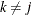. This particular substitution pattern might be too restrictive in some choice settings.

The IIA property of the conditional logit model follows from the assumption that the random components of utility are identically and independently distributed. The other models in PROC MDC (namely, nested logit, HEV, mixed logit, and multinomial probit) relax the IIA property in different ways.

For an example of Hausman’s specification test of IIA assumption, see Hausman’s Specification Test.